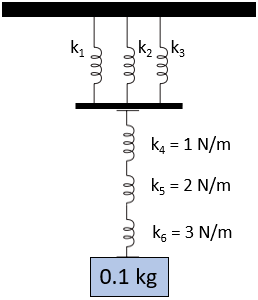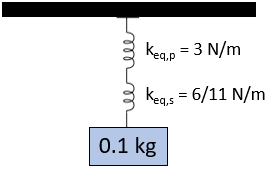## Compounding Springs

Consider a spring system is attached to a ceiling as shown in the figure. Springs 1, 2, and 3 are arranged in parallel, and each has a spring constant of 1 N/m. Springs 4, 5, and 6 are arranged in series. If a 0.1 kg mass is attached to the open end of the spring system, how many meters will the spring system stretch in total?Hint
The spring constant for springs in parallel:
$$k_{eq}=\sum_{i} k_i$$$Hint 2 The spring constant for springs in series: $$\frac{1}{k_{eq}}=\sum_{i}\frac{1}{k_i}$$$
We’ll need to determine the equivalent spring constant of the entire system, which can be broken down into two parts. Let’s first analyze only the springs in parallel. The spring constant for springs in parallel:
$$k_{eq}=\sum_{i} k_i$$$Since the problem statement has three springs in parallel: $$k_{eq,p}=k_1+k_2+k_3=1\frac{N}{m}+1\frac{N}{m}+1\frac{N}{m}=3\:N/m$$$
Next, let’s analyze the springs in series. The spring constant for springs in series:
$$\frac{1}{k_{eq}}=\sum_{i}\frac{1}{k_i}$$$Since the problem statement has three springs in series: $$\frac{1}{k_{eq,s}}=\frac{1}{k_4}+\frac{1}{k_5}+\frac{1}{k_6}=\frac{1}{1N/m}+\frac{1}{2N/m}+\frac{1}{3N/m}$$$
$$\frac{1}{k_{eq,s}}=\frac{6}{6N/m}+\frac{3}{6N/m}+\frac{2}{6N/m}=\frac{11m}{6N}$$$$$\frac{1}{\frac{1}{k_{eq,s}}}=\frac{1}{\frac{11m}{6N}}$$$
$$k_{eq,s}=\frac{6}{11}\:N/m$$$Based on the two spring constant equivalents we just calculated, we can simplify the problem graphic by treating the new system as two springs in series:$$\frac{1}{k_{eq}}=\frac{1}{k_{eq,p}}+\frac{1}{k_{eq,s}}=\frac{1}{3N/m}+\frac{11}{6N/m}$$$
$$\frac{1}{k_{eq}}=\frac{2}{6N/m}+\frac{11}{6N/m}=\frac{13m}{6N}$$$$$\frac{1}{\frac{1}{k_{eq}}}=\frac{1}{\frac{13m}{6N}}$$$
$$k_{eq}=\frac{6}{13}\:N/m$$$A spring’s deflection and force are related by: $$F_s=k\delta$$$
where $$F_s$$ is the forced applied to the spring, $$k$$ is the spring constant, and $$\delta$$ is the change in spring length from the un-deformed spring length.
Before proceeding, we need to determine the spring force by multiplying the mass by gravity:
$$F_s=mass\times acceleration=(0.1kg)(9.8m/s^2)=0.98\:N$$$Now our units should cancel out when we solve for the spring’s stretched distance: $$\delta=\frac{F_s}{k_{eq}}=\frac{0.98N\cdot 13m}{6N}=2.1\:m$$$
2.1 m